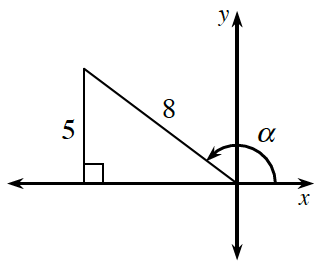### Home > PC3 > Chapter 7 > Lesson 7.1.3 > Problem7-42

7-42.

Use the diagram at right to calculate the exact values of the following trigonometric expressions.

1. $\sin(α)$

Use the values given in the diagram.

2. $\cos(α)$

Calculate the length of the base of the triangle. What should the sign be?

3. $\tan(α)$

Use the information in the triangle and your calculated base from part (b).